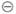Chapter 8.1, Problem 12ESMathematical Excursions (MindTap C...

4th Edition
Richard N. Aufmann + 3 others
ISBN: 9781305965584

Solutions

Chapter
SectionMathematical Excursions (MindTap C...

4th Edition
Richard N. Aufmann + 3 others
ISBN: 9781305965584
Textbook Problem

Evaluate each expression, where ⊕ and ⊖ indicate addition and subtraction, respectively, using a 12-hour clock.5 ⊖ 7

To determine

The subtraction 57 on a 12-hour clock.

Explanation

Given information: denote the subtraction on 12-hour.

Calculation:

57 denote the time 7 hours ago 5 o’clock...

Still sussing out bartleby?

Check out a sample textbook solution.

See a sample solution

The Solution to Your Study Problems

Bartleby provides explanations to thousands of textbook problems written by our experts, many with advanced degrees!

Get Started

In problems 45-48, determine a value for that makes each statement true. 47.

Mathematical Applications for the Management, Life, and Social Sciences

Let f(x) = 2x2 x + 1. Find: a. f(x 1) + f(x + 1) b. f(x + 2h)

Applied Calculus for the Managerial, Life, and Social Sciences: A Brief Approach

Determine the infinite limit. limx0+(1xlnx)

Single Variable Calculus: Early Transcendentals, Volume I

If STTV, explain why STV is an isosceles triangle.

Elementary Geometry For College Students, 7e

. 16.Minimize Subject to C=2x+3y x+y10x+2y122x+y12x0,y0

Finite Mathematics for the Managerial, Life, and Social Sciences